Get instant live expert help with Excel or Google Sheets“My Excelchat expert helped me in less than 20 minutes, saving me what would have been 5 hours of work!”

#### Post your problem and you’ll get expert help in seconds.

Your message must be at least 40 characters
Our professional experts are available now. Your privacy is guaranteed.

# Data validation allow weekday only

We can use the Excel WEEKDAY function to ensure that data validation allows weekday only. The steps below will walk through the process of using Data Validation to allow weekday only.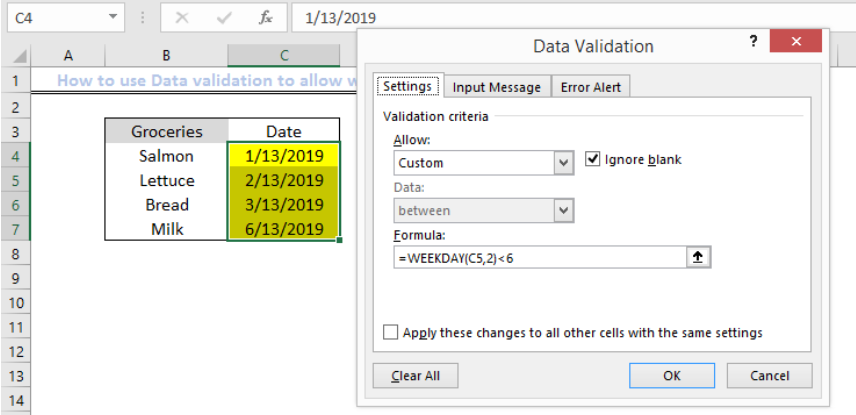Figure 1 – Example of Data Validation to allow weekday only

## General Formula

`=WEEKDAY(A1,2)<6`

## Formula

`=WEEKDAY(C5,2)<6`

## Setting up the Data

• We will set up our data in Columns B and C
• Column B will contain our Groceries
• Column C will contain our dates of purchase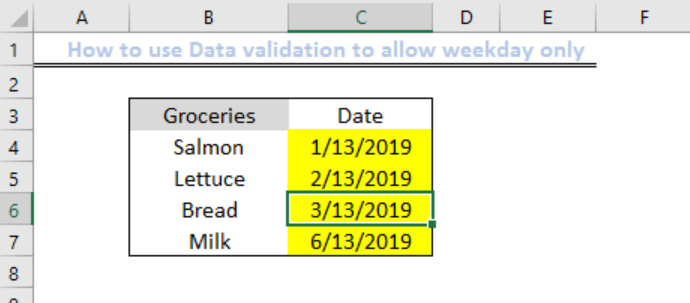Figure 2 – Setting up the Data

## Applying the WEEKDAY customized function

• We will highlight Cells C5 to C7
• We will click on Data Validation in the Data tab within the ribbon at the top of the worksheet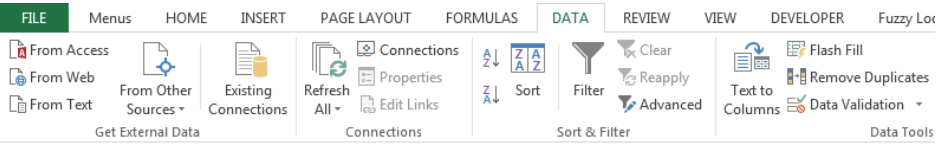Figure 3 – Clicking on Data tab

• We will check the ignore blank box, select custom, and enter the formula below in the formula box
`=WEEKDAY(C5,2)<6`
• We will press OK
• This will allow weekdays only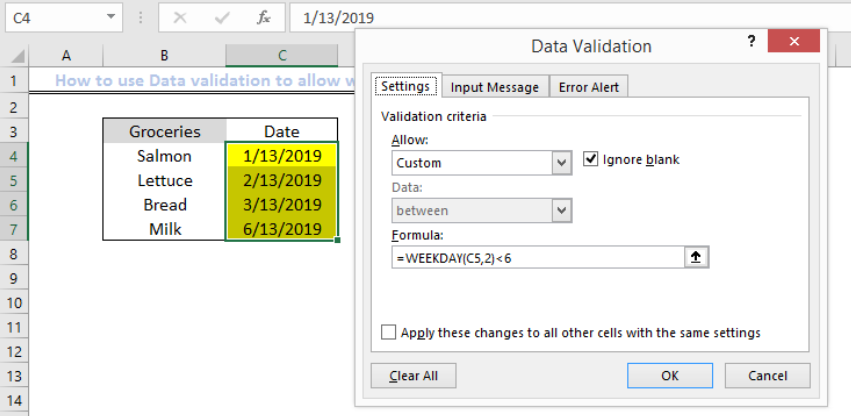Figure 4 – Applying the WEEKDAY customized function

## An Alternative Formula for Data validation

We can also create a return value argument that would allow the dates from 1 (Sunday) to 7 (Saturday).

• We will again highlight on Cells C5 to C7 and select Data Validation
• We will select custom, and enter this formula below in the formula dialog box
`=AND(WEEKDAY(B2)<>1,WEEKDAY(B2)<>7)`
• We will press OK. This would return TRUE for all values if they fall within Saturday to Sunday.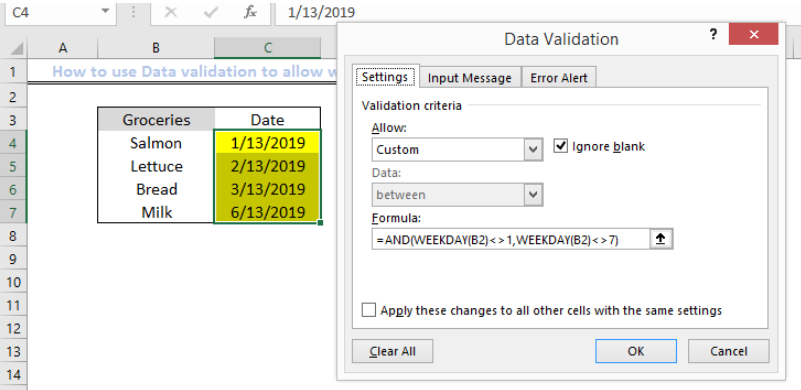Figure 5 – Alternative formula for Data Validation

## Explanation

We use the Data validation rules to restrict our cells so any user can only add values within the specific restriction. For our formula, the return value, 2 is a Monday-based number. This would be compared by Excel against 6. Therefore, any values less than 6 will return true. If the value is more than 6, the date is Sunday or Saturday, then validation fails.

## Note

• If we wish to allow only dates that occur in the weekend such as Sunday or Saturday, then we can use this formula:
`=WEEKDAY(C5,2)>5`
• The Cell reference in the data validation formulas are always relative to the upper left cell, hence the reason our formula contains, C5.

## Instant Connection to an Expert through our Excelchat Service

Most of the time, the problem you will need to solve will be more complex than a simple application of a formula or function. If you want to save hours of research and frustration, try our live Excelchat service! Our Excel Experts are available 24/7 to answer any Excel question you may have. We guarantee a connection within 30 seconds and a customized solution within 20 minutes.

### Did this post not answer your question? Get a solution from connecting with the expert.Another blog reader asked this question today on Excelchat:
Solution examplesI want to be able to alter the appearance (font color and fill) of a column of numbers using conditional formatting, based on being greater than or less than the numbers from another column. I am using Excel 2016. I am unable to get any formula to work. Please help!
Solved by I. J. in 59 minsI need assistance with an excel formula. I have a 12 month sheet that has a summary page on the 13 page. the monthly totals changes so on the summary page I want to sum the difference - eg. if Jan total is 1500, feb total is 1000, mar total is 800, I want the summary page to show the current amount of 800 when you place the formula to capture all months---showing the diminishing value rather than the sum of all the pages
Solved by E. L. in 60 minsI need a range of numbers to become the number in the next column
Solved by D. F. in 47 minsUse the Vlookup Function to complete the "employee" column of table 2. Use "job Id" from table 2 as your lookup_value(s) and table 1 as your reference.
Solved by C. H. in 16 minsI need to count number of dates from a timestamped date in column from the completed date column to show the number of days. I have not successfully been able to do this. I am a novice Excel user. Can you help?
Solved by O. B. in 15 mins## Subscribe to Excelchat.coAnother blog reader asked this question today on Excelchat: